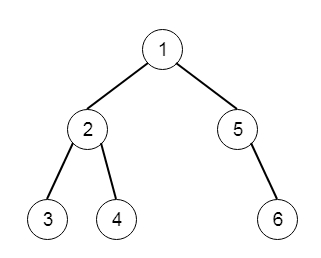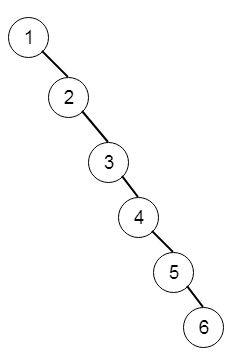# Flatten Binary Tree to Linked List in C++

Suppose we have a binary tree; we have to flatten it into linked list in place. So if the tree is like −The output tree will be −To solve this, we will follow these steps −

• ser prev := null

• Define a recursive function solve(), that will take root as input.

• if root is null, then return

• solve(right of root)

• solve(left of root)

• right of root := prev, left of root := null

• prev := root

Let us see the following implementation to get better understanding −

## Example

Live Demo

#include <bits/stdc++.h>
using namespace std;
class TreeNode{
public:
int val;
TreeNode *left, *right;
TreeNode(int data){
val = data;
left = NULL;
right = NULL;
}
};
void insert(TreeNode **root, int val){
queue<TreeNode*> q;
q.push(*root);
while(q.size()){
TreeNode *temp = q.front();
q.pop();
if(!temp->left){
if(val != NULL)
temp->left = new TreeNode(val);
else
temp->left = new TreeNode(0);
return;
}else{
q.push(temp->left);
}
if(!temp->right){
if(val != NULL)
temp->right = new TreeNode(val);
else
temp->right = new TreeNode(0);
return;
}else{
q.push(temp->right);
}
}
}
TreeNode *make_tree(vector<int> v){
TreeNode *root = new TreeNode(v);
for(int i = 1; i<v.size(); i++){
insert(&root, v[i]);
}
return root;
}
class Solution {
public:
TreeNode* prev = NULL;
void flatten(TreeNode* root) {
if(!root) return;
flatten(root->right);
flatten(root->left);
root->right = prev;
root->left = NULL;
prev = root;
}
};
main(){
vector<int> v = {1,2,5,3,4};
TreeNode *root = make_tree(v);
Solution ob;
(ob.flatten(root));
TreeNode *ptr = root;
while(ptr != NULL && ptr->val != 0){
cout << ptr->val << ", ";
ptr = ptr->right;
}
}

## Input

[1,2,5,3,4]

## Output

1, 2, 3, 4, 5,